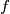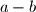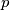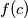### IMO Shortlist 2009 problem N3

Kvaliteta:
Avg: 4,0
Težina:
Avg: 7,0
Let$f$ be a non-constant function from the set of positive integers into the set of positive integer, such that$a-b$ divides$f\!\left(a\right)-f\!\left(b\right)$ for all distinct positive integers$a$,$b$. Prove that there exist infinitely many primes$p$ such that$p$ divides$f\!\left(c\right)$ for some positive integer$c$.

Proposed by Juhan Aru, Estonia
Izvor: Međunarodna matematička olimpijada, shortlist 2009